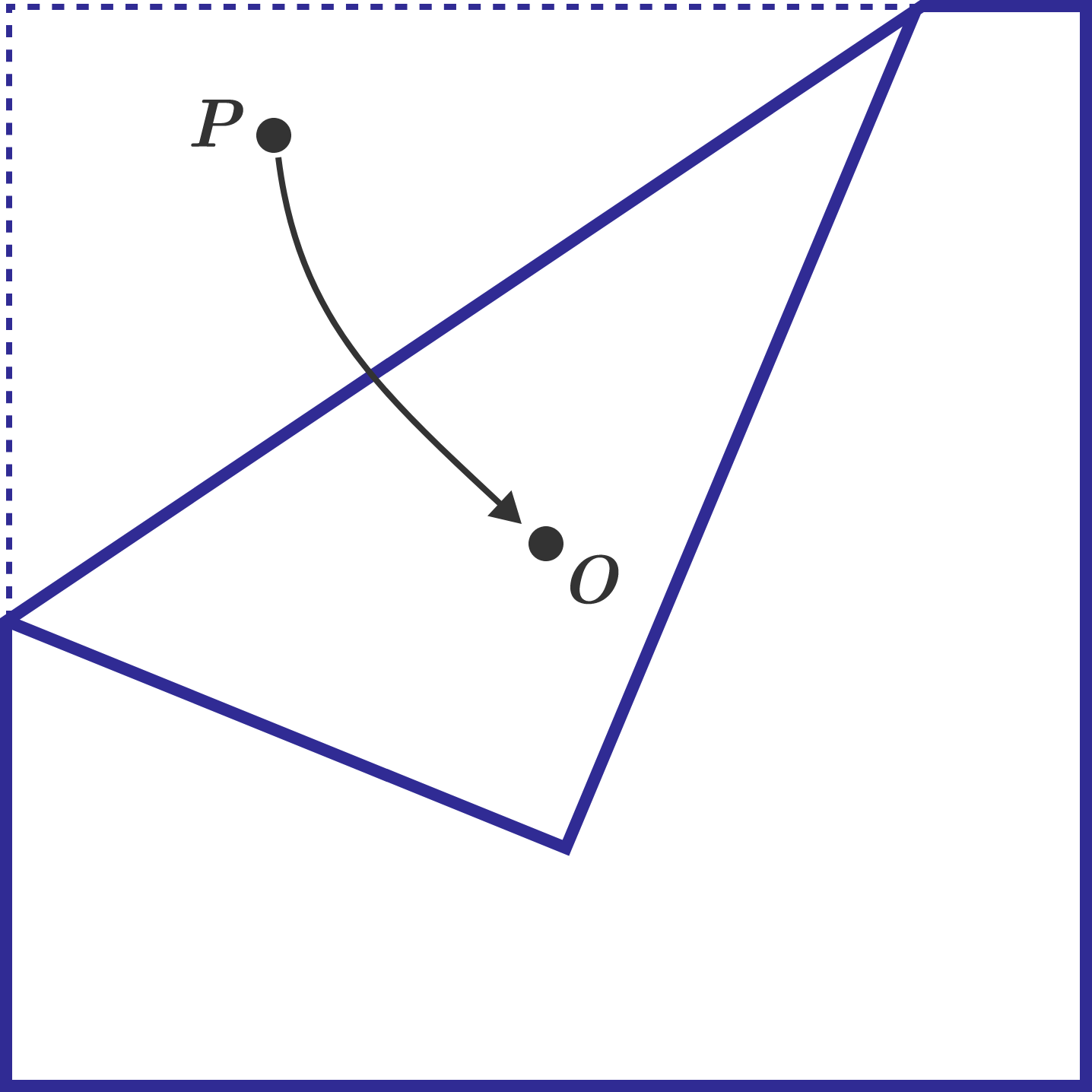# Origami Probability

Geometry Level 4A square sheet of paper has its center marked with point $$O$$. A random point is uniformly chosen on the square paper, and this point is labeled $$P$$. The paper is then folded so that point $$P$$ coincides with point $$O$$. Let the probability that a pentagon is formed after the fold be $$N$$. Find the value of $$\lfloor 1000N \rfloor$$.

×